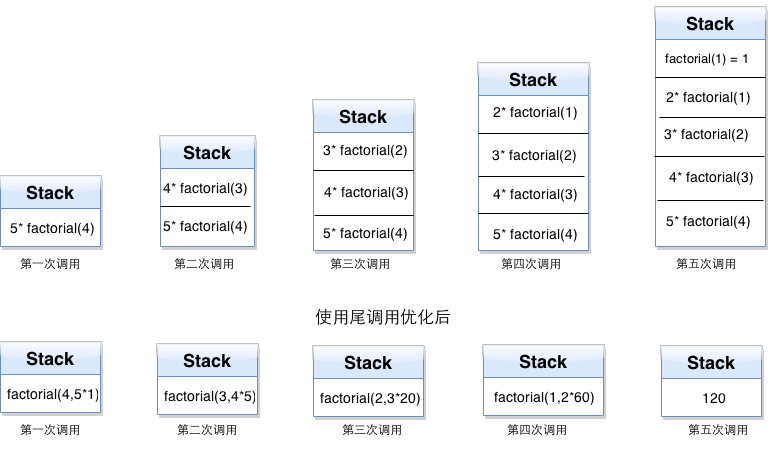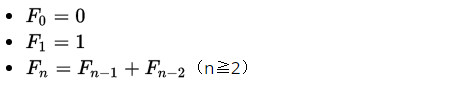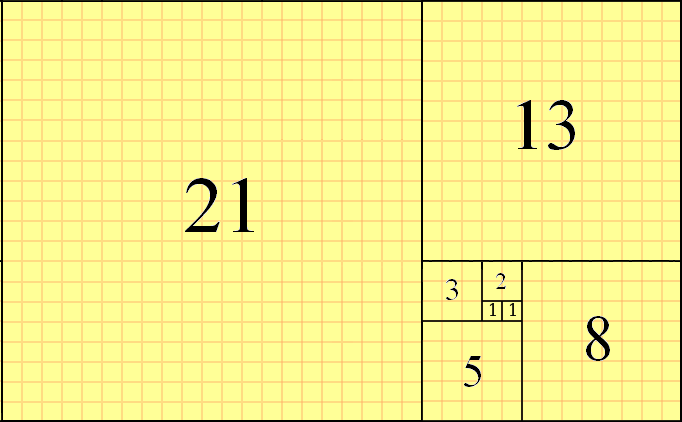October 24, 2016

# ES6函数与Lambda演算

repository-tree

• ES6
• Vue.js
• Webpack
• Vuex
• lodash
• GitHub API

## # 探索发现之旅

### # 高阶函数

mapfilterreduce 均属于高阶函数，高阶函数并不神秘，我们日常编程也会用到。

ES6 中的 `map` 例子

``````const arr = [1, 2, 3, 4, 5, 6]

const powArr = arr.map(v => v * v)

console.log(powArr) // [ 1, 4, 9, 16, 25, 36 ]
``````
1
2
3
4
5

### # 尾调用

``````function f(x) {
return g(x);
}
``````
1
2
3

### # 尾递归

``````// ES5
function factorial(n) {
return n === 1 ? 1 : n * factorial(n - 1);
}

factorial(6) // => 720

// ES6
const factorial = n => n === 1 ? 1 : n * factorial(n - 1)

factorial(6) // => 720
``````
1
2
3
4
5
6
7
8
9
10
11

• 调用自身函数(Self-called)；
• 计算仅占用常量栈空间(Stack Space)。

``````// ES5
function factorial(n, total) {
return n === 1 ? total : factorial(n - 1, n * total);
}

factorial(6, 1) // => 720

// ES6
const factorial = (n, total) => n === 1 ? total : factorial(n - 1, n * total)

factorial(6, 1) // => 720
``````
1
2
3
4
5
6
7
8
9
10
11### # 柯里化

``````// 阶乘尾递归优化写法
function currying(fn, n) {
return function (m) {
return fn.call(this, m, n);
};
}

function tailFactorial(n, total) {
if (n === 1) return total;
return tailFactorial(n - 1, n * total);
}

const factorial = currying(tailFactorial, 1);

factorial(6) // => 720
``````
1
2
3
4
5
6
7
8
9
10
11
12
13
14
15

``````const fact = (n, total) => n === 1 ? total : fact(n - 1, n * total)

const currying = f => n => m => f(m, n)

const factorial = currying(fact)(1)

factorial(6) // => 720
``````
1
2
3
4
5
6
7

``````const fact = (n, total = 1) => n === 1 ? total : fact(n - 1, n * total)

factorial(6) // => 720
``````
1
2
3

### # Lambda表达式

`JavaScript` 中，Lambda表达式可以表示匿名函数。

``````// ES5
var f = function (x) {
return x;
};

// ES6
const f = x => x
``````
1
2
3
4
5
6
7

`lambda表达式` 来写是这样子的：`λx.x`

``````// ES5
function factorial(f, n) {
return n === 1 ? 1 : n * f(f, n - 1)
}

factorial(factorial, 6) // => 720

// ES6
const factorial = (f, n) => n === 1 ? 1 : n * f(f, n - 1)

factorial(factorial, 6) // => 720
``````
1
2
3
4
5
6
7
8
9
10
11

``````const fact = (f, n ,total = 1) => n === 1 ? total : f(f, n - 1, n * total)

const currying = f => n => m => f(f, m ,n)

const factorial = currying(fact)()

factorial(6) // => 720
``````
1
2
3
4
5
6
7

### # Lambda演算

Lambda演算中的所有函数都是匿名的，它们没有名称，它们只接受一个输入变量，即独参函数。

`const invokeWithSelf = f => f(f)`

``````const fact = f => (total = 1) => n => n === 1 ? total : f(f)(n * total)(n - 1)

const factorial = fact(fact)()

factorial(6) // => 720
``````
1
2
3
4
5

### # 黑魔法Y组合子

`Y = λf.(λx.f(xx))(λx.f(xx))`

η-变换后的写法：

`Y = λf.(λx.f(λv.x(x)(v)))(λx.f(λv.x(x)(v)))`

``````const Y = f =>
(x => f(v => x(x)(v)))
(x => f(v => x(x)(v)))
``````
1
2
3

### # Y组合子推导

#### # 以匿名函数递归开始

``````const fact = f => (total = 1) => n => n === 1 ? total : f(f)(n * total)(n - 1)

const factorial = fact(fact)()

factorial(6) // => 720
``````
1
2
3
4
5

``````const fact = f =>
(g => (total = 1) => n => n === 1 ? total : g(n * total)(n - 1))(f(f))

const factorial = fact(fact)()

factorial(6) // => Maximum call stack size exceeded
``````
1
2
3
4
5
6

#### # η-变换

lambda演算中有效的η-变换`f = λx.(fx)`

JavaScript中的η-变换`f = x => f(x)`

``````const fact = x => (f => (total = 1) => n => n === 1 ? total : f(n * total)(n - 1))(v => x(x)(v))

const factorial = fact(fact)()

factorial(6) // => 720
``````
1
2
3
4
5

#### # 抽离共性

``````const fact = f => (total = 1) => n => n === 1 ? total : f(n * total)(n - 1)

const factorial = (x => fact((v => x(x)(v))))(x => fact((v => x(x)(v))))()

factorial(6) // => 720
``````
1
2
3
4
5

#### # 构建Y

``````const fact = f => (total = 1) => n => n === 1 ? total : f(n * total)(n - 1)

const Y = f => (x => f(v => x(x)(v)))
(x => f(v => x(x)(v))) // 瞧，这不就是黑魔法Y组合子嘛

const factorial = Y(fact)()

factorial(6) // => 720
``````
1
2
3
4
5
6
7
8

## # 沿途风景

### # 斐波那契数列`0,1,1,2,3,5,8,13,21,34,55,89,144,233......```````// 非尾递归
function fibonacci (n) {
if ( n <= 1 ) return 1;
return fibonacci(n - 1) + fibonacci(n - 2);
}

fibonacci(6) // 13
``````
1
2
3
4
5
6
7

``````// 尾递归写法
function fibonacci (n , before , after) {
if( n <= 1 ) return before;
return fibonacci (n - 1, after, before + after);
}

fibonacci(6, 1, 2) // 13
``````
1
2
3
4
5
6
7

``````// ES6 lambda calculus
const Y = f => (x => f(v => x(x)(v)))(x => f(v => x(x)(v)))

const fibonacci = Y(
f => (n) => n <= 1 ? 1 : f(n - 1) + f(n - 2)
)

fibonacci(6) // 13
``````
1
2
3
4
5
6
7
8

### # 德罗斯特效应## # 参考

Lambda演算

JS 函数式编程指南

《ECMAScript 6 入门》